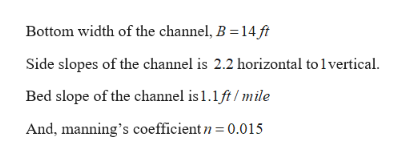# Water flows uniformly in a trapezoidal channel with a 14-ft-wide bed and 2.2:1 (m = 2.2) side slopes. If the bed slope is 1.1 ft/mile and n = 0.015, find the flow rate when the depth is 9 ft.

Question
3 views

Water flows uniformly in a trapezoidal channel with a 14-ft-wide bed and 2.2:1 (m = 2.2) side slopes. If the bed slope is 1.1 ft/mile and n = 0.015, find the flow rate when the depth is 9 ft.

check_circle

Step 1

Given information:help_outlineImage TranscriptioncloseBottom width of the channel, B =14 ft Side slopes of the channel is 2.2 horizontal to lvertical. Bed slope of the channel is 1.1ft/mile And, manning's coefficientn 0.015 fullscreen
Step 2

To determine:

Step 3

Calculation:

From the given data draw the ch...

### Want to see the full answer?

See Solution

#### Want to see this answer and more?

Solutions are written by subject experts who are available 24/7. Questions are typically answered within 1 hour.*

See Solution
*Response times may vary by subject and question.
Tagged in

### Fluid Mechanics